Drop a Query

# Time Series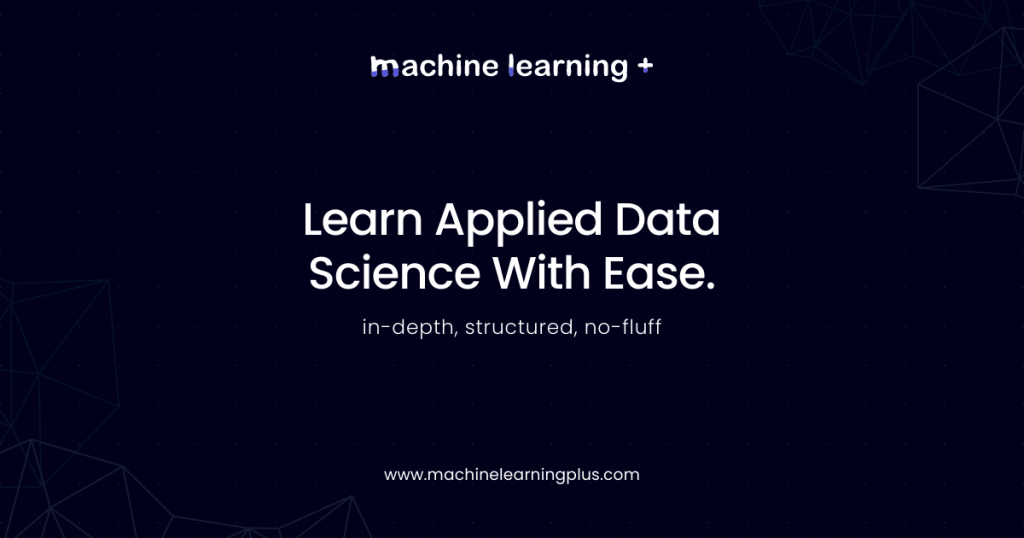## Granger Causality Test

Introduced in 1969 by Clive Granger, Granger causality test is a statistical test that is used to determine if a particular time series is helpful in forecasting another series. What is Granger Causality? Let’s first understand when is the Granger causality test is useful. Let’s say you have two time series data X and Y, …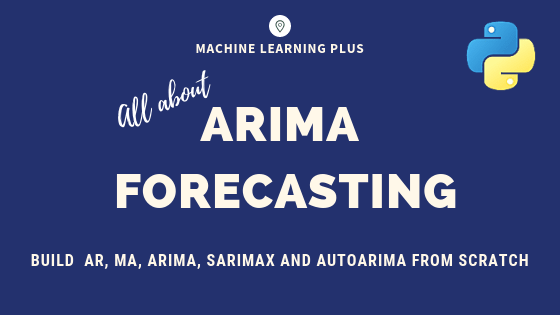## ARIMA Model – Complete Guide to Time Series Forecasting in Python

Using ARIMA model, you can forecast a time series using the series past values. In this post, we build an optimal ARIMA model from scratch and extend it to Seasonal ARIMA (SARIMA) and SARIMAX models. You will also see how to build autoarima models in python ARIMA Model – Time Series Forecasting. Photo by Cerquiera …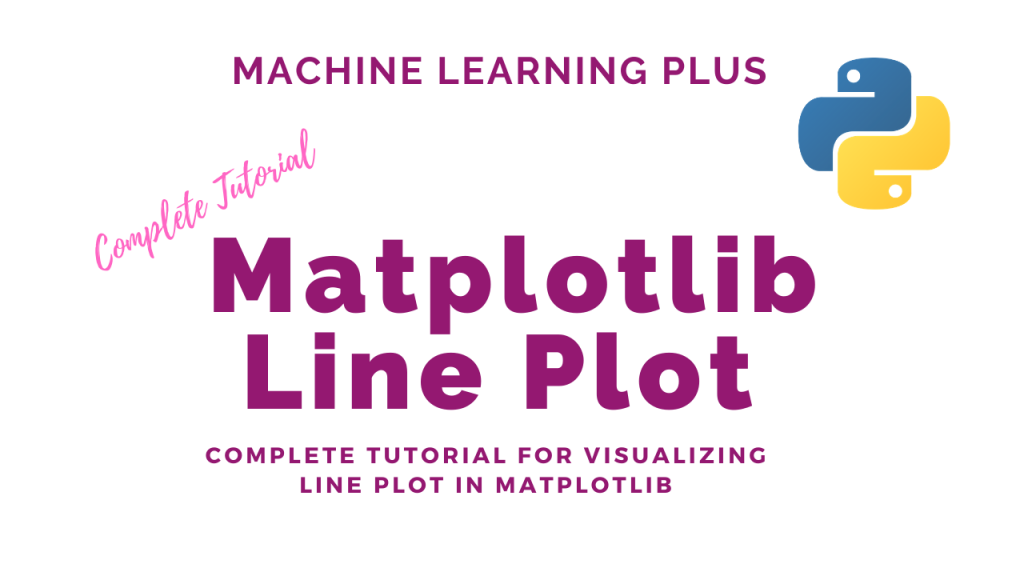## Matplotlib Line Plot – How to create a line plot to visualize the trend?

Line plot is a type of chart that displays information as a series of data points connected by straight line segments. A line plot is often the first plot of choice to visualize any time series data. Contents What is line plot? Simple Line Plot Multiple Line Plot in the same graph Creating a secondary …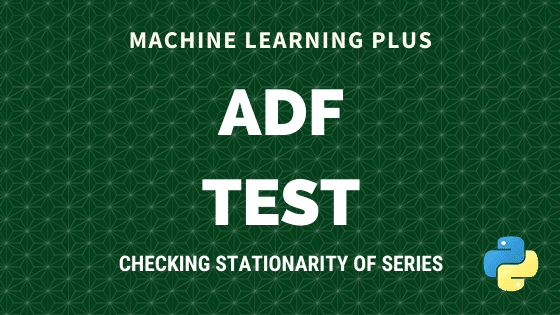Augmented Dickey Fuller test (ADF Test) is a common statistical test used to test whether a given Time series is stationary or not. It is one of the most commonly used statistical test when it comes to analyzing the stationary of a series. 1. Introduction In ARIMA time series forecasting, the first step is to …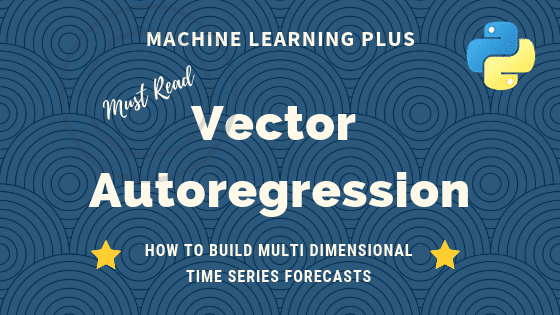## Vector Autoregression (VAR) – Comprehensive Guide with Examples in Python

Vector Autoregression (VAR) is a forecasting algorithm that can be used when two or more time series influence each other. That is, the relationship between the time series involved is bi-directional. In this post, we will see the concepts, intuition behind VAR models and see a comprehensive and correct method to train and forecast VAR …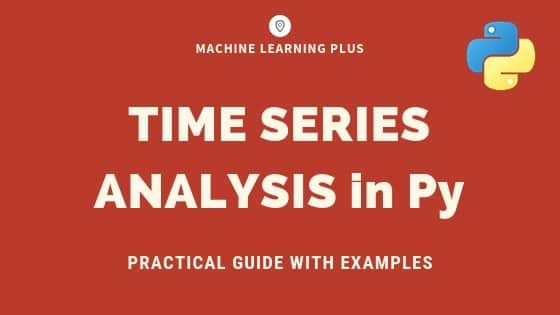## Time Series Analysis in Python – A Comprehensive Guide with Examples

Time series is a sequence of observations recorded at regular time intervals. This guide walks you through the process of analyzing the characteristics of a given time series in python. Time Series Analysis in Python – A Comprehensive Guide. Photo by Daniel Ferrandiz. Contents What is a Time Series? How to import Time Series in …Course Preview

## Machine Learning A-Z™: Hands-On Python & R In Data Science

### Free Sample Videos:#### Machine Learning A-Z™: Hands-On Python & R In Data Science#### Machine Learning A-Z™: Hands-On Python & R In Data Science#### Machine Learning A-Z™: Hands-On Python & R In Data Science#### Machine Learning A-Z™: Hands-On Python & R In Data Science